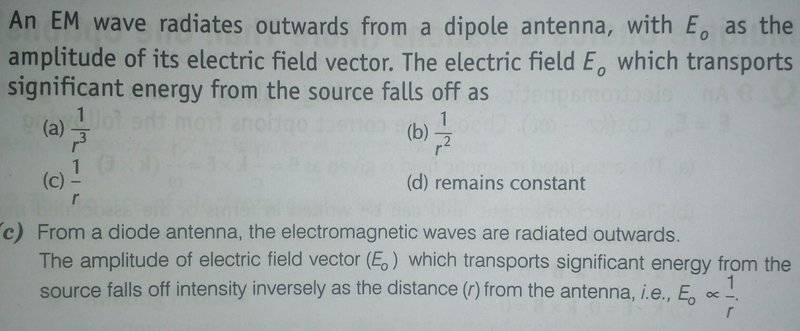# Electric field varying with distance

Jahnavi
[Moderator note: moved from general physics, no template.]This is a solved example given in the book . Could someone help me understand how amplitude of electric field has an inverse relationship with distance ?

Only the very basics of EM waves are covered in the book so I would appreciate if someone could explain in a simple language .

Thank you .

Last edited:

## Answers and Replies

Homework Helper
Gold Member
For a dipole antenna, the electric field of the radiated wave will stay parallel to the antenna dipole. This means the radiation pattern will mostly be 2 dimensional as a circle, centered at the transmitting antenna. As the circumference increases the field strength is "spread out" over more circumference.
So the electric field strength is divided by the length of the circumference. The circumference of a circle is proportional to r.

RedDelicious
Recall that the irradiance (also called intensity) falls off as 1/r^2 (just think of the flux through spherical surfaces centered on the source) and is also proportional to E^2 (shown in just about every book, even if not derived). Putting both together,

$$I \propto 1/r^2 \propto E^2 => E \propto 1/r$$

•Jahnavi
Jahnavi
Recall that the irradiance (also called intensity) falls off as 1/r^2 (just think of the flux through spherical surfaces centered on the source) and is also proportional to E^2 (shown in just about every book, even if not derived). Putting both together,

$$I \propto 1/r^2 \propto E^2 => E \propto 1/r$$

Thanks !